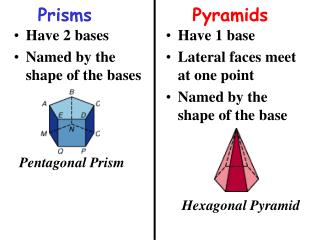DownloadDownload PresentationPrisms

# Prisms

Télécharger la présentation## Prisms

- - - - - - - - - - - - - - - - - - - - - - - - - - - E N D - - - - - - - - - - - - - - - - - - - - - - - - - - -
##### Presentation Transcript

1. Have 2 bases Named by the shape of the bases Have 1 base Lateral faces meet at one point Named by the shape of the base Prisms Pyramids Pentagonal Prism Hexagonal Pyramid

2. Prisms BASE HEIGHT BASE

3. Right Prism Oblique Prism height

4. cylinder Base Lateral Surface Height Base

5. Lateral Area (LA): the sum of the areas of the solid’s lateral surfaces Total Surface Area (TA or SA): the sum of the areas of all of its surfaces P = PERIMETER of base (add all sides of the base) B = area of BASE (use different formulas according to the shape of the base) h = HEIGHT of the solid

6. Formulas for PRISMS LA = PhLateral Area = Perimeter of Base  height of prism SA = Ph + 2BSurface Area = Perimeter of Base  height of prism + 2  Area of Base

7. EX:1 Find the lateral area.(One base is the side that is on the ground.) 2.1 cm 3.6 cm 6.8 cm LA = Ph LA = 20.8 x 2.1 LA = 43.68 cm2

8. EX:2 Find the surface area. 2.1 cm 3.6 cm 6.8 cm SA = Ph + 2B SA = 43.68 + 2(24.48) SA = 92.64 cm2

9. EX:3 Find the lateral area.(One base is the side that is on the ground.) LA = Ph 6 m LA = 24 x 9 8 m LA = 216 m2 9 m

10. EX:4 Find the surface area.(One base is the side that is on the ground.) SA = Ph + 2B 6 m SA = 216 + 2(24) 8 m SA = 264 m2 9 m

11. Formulas for Cylinders LA = 2rh SA = 2rh + 2r2

12. EX:6 Find the surface area. Round to the nearest tenth. 5 cm SA = 2rh + 2r2 3.8 cm

13. Surface Area of Pyramids and ConesSection 12.3 Goal – to find the surface area of a pyramid and the surface area of a cone

14. Pyramids are classified by the shapes of their bases

15. Surface Area verses Lateral Area of a Pyramid The lateral area of a pyramid: LA = P = Perimeter of the base l = slant height The surface area of a pyramid: SA = B = Area of the base

16. Example

17. Surface Area verses Lateral Area of a Cone The lateral area of a cone: LA = r = radius of the base l= slant height The surface area of a cone: SA =

18. Example

19. Students

20. Students

21. Students